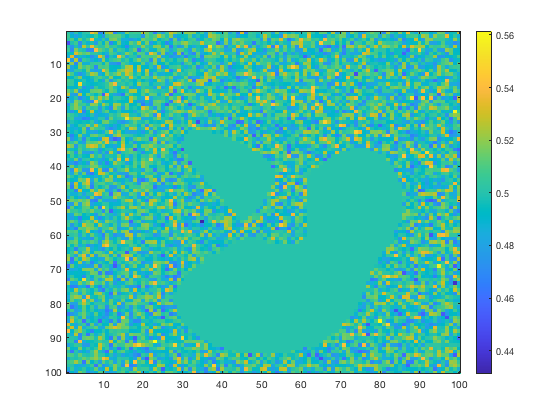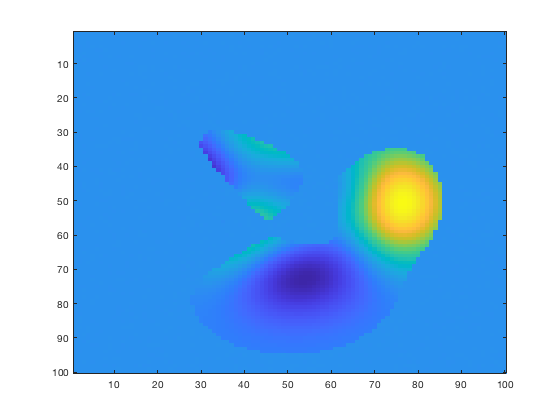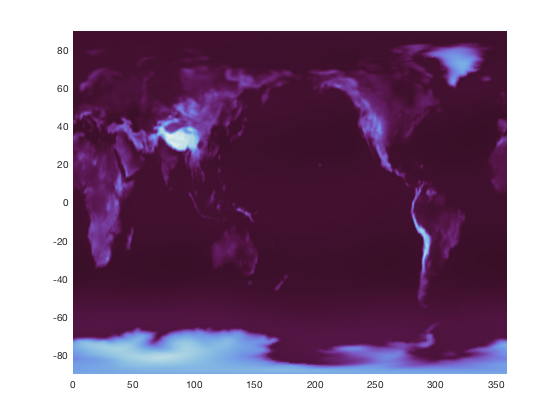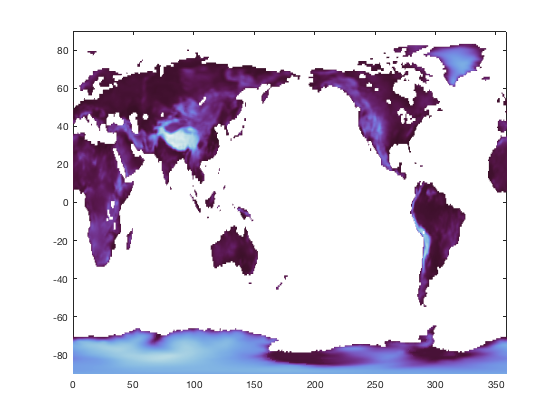Back to Climate Data Tools Contents

## Syntax

```Am = mask3(A,mask)

## Description

Am = mask3(A,mask) sets all elements along the third dimenion of the 3D matrix A to NaN wherevever there are true elements in the corresponding 2D logical mask.

## Example 1: Set masked values to NaN

Here's some random sample data A which contains 300 time steps of a 100x100 grid. Make a mask and set all the true values in the mask to NaN:

```% Gridded time series:
A = rand(100,100,300);

% true wherever sample peaks data exceeds 1:

% Make A NaN where the mask is true:

% Plot:
figure
subplot(1,2,1)
colorbar
axis image

subplot(1,2,2)
imagesc(sum(isfinite(Am),3))
colorbar
title 'number of finite values in Am'
axis image
```## Example 2: Set masked values to a scalar

Suppose instead you want to set all the masked grid cells to a specific value, like 0.5. Here's how you'd do that:

```Am = mask3(A,mask,0.5);

figure
imagesc(mean(Am,3))
colorbar
```In the figure above we see that all the true values in the mask are exactly 0.5. Everything else looks like noise because all the other grid cells show the average of 300 random values.

## Example 3: Fill masked regions with a grid

Sometimes when you have a gridded time series you want to fill a region not with a single scalar value, but with a corresponding grid of values. For example, for the same mask as above, you might have a grid of replacement values repgrid like this:

```repgrid = rot90(peaks(100),1);

figure
subplot(1,2,1)
axis image

subplot(1,2,2)
imagesc(repgrid)
axis image
title 'this is the replacement grid'
```Replace all the masked grid cells in A with the corresponding values in repgrid:

```Am = mask3(A,mask,repgrid);

figure
imagesc(mean(Am,3))
```## Example 4: Surface pressure time series

This example uses data frome the ERA_Interim_2017.nc dataset. (For more on working with .nc files, see the NetCDF Tutorial.) Start by loading the data:

```filename = 'ERA_Interim_2017.nc';
```

The surface pressure time series SP has the dimensions

```size(SP)
```
```ans =
480   241    12
```

which correspond to latitude, longitude, and time.

Let's mask out all the points in SP that correspond to ocean. To do that, make a Lat,Lon grid from the 1d lat,lon arrays and use island to find out which grid cells are land and which ones are ocean.

```[Lat,Lon] = meshgrid(lat,lon);

land = island(Lat,Lon);

% ocean is *not* land:
ocean = ~land;
```

Here's the time-averaged mean of the full dataset:

```figure
pcolor(Lon,Lat,mean(SP,3))
axis tight
cmocean dense % optional colormap
```Now set all ocean grid cells to NaN:

```SPm = mask3(SP,ocean);

figure
pcolor(Lon,Lat,mean(SPm,3))